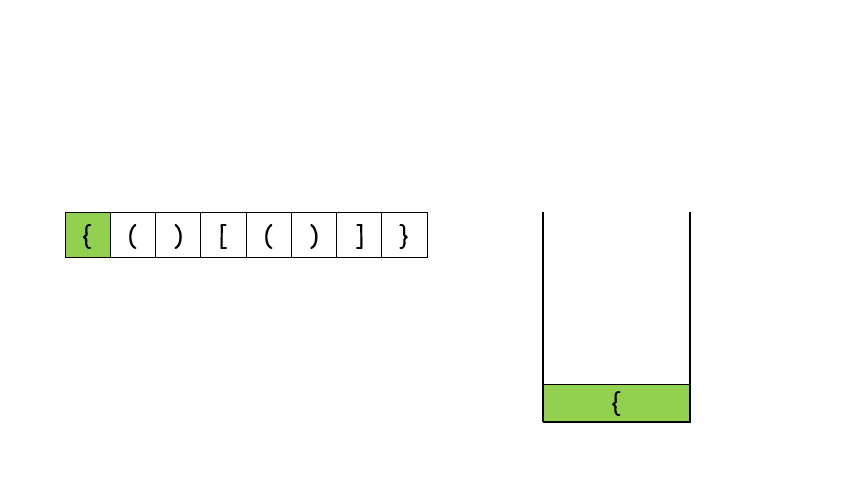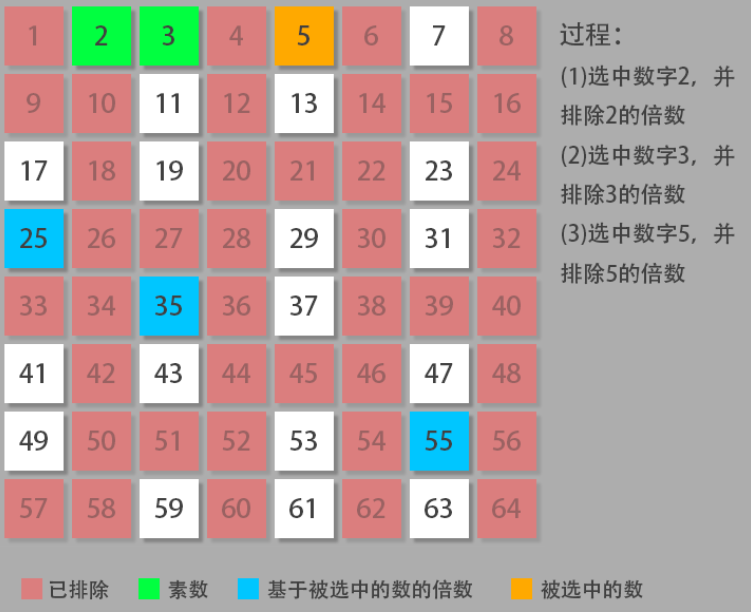# LeetCode刷题记录

Posted by kevin on July 1, 2020

## 1 两数之和

class Solution {	// 我写的辣鸡暴力法
public:
vector<int> twoSum(vector<int>& nums, int target) {
vector<int> result;
for (int i = 0; i < nums.size(); ++i) {
int t1 = nums[i];
for (int j = i + 1; j < nums.size(); ++j) {
int t2 = nums[j];
if (t1 + t2 == target) {
result.push_back(i);
result.push_back(j);
}
}
}
return result;
}
};


class Solution {
public:
vector<int> twoSum(vector<int>& nums, int target) {
map<int,int> a;//提供一对一的hash
vector<int> b(2,-1);//用来承载结果，初始化一个大小为2，值为-1的容器b
for(int i=0;i<nums.size();i++)
{
if(a.count(target-nums[i])>0)   //找到了目标
{
b=a[target-nums[i]];
b=i;
break;
}
a[nums[i]]=i;//反过来放入map中，用来获取结果下标
}
return b;
};
};


## 20 有效的括号

左括号必须用相同类型的右括号闭合。class Solution {
public:
bool isValid(string s) {
/*
1. 首先设定哈希表,依次保存三个开括号(分别对应1,2,3)与三个闭括号(分别对应4,5,6),以及栈(只放入开括号,遇到对应闭括号,则出栈)还有最后一个正确bool值,判断是否正确(比如第一个就是闭括号,必然错误),且默认为真
2. for遍历string字符串
1. 如果为开括号,入栈
2. 否则栈非空时,且接下来的为对应闭括号,则出栈
3. 否则(此时隐含表达为'这是个闭括号'),则bool值为假
3. 如果栈非空时,则说明闭括号少了,bool为假
4. 返回bool值
*/
//哈希表,存储,以及栈
//傻逼博客不在一起会报错，我只能这样搞，看得懂就行
data = {'(',1},{'[',2},{'{',3},{')',4},{']',5},{'}',6}
unordered_map<char,int> m{data};
stack <char> st;
//用来判断如果第一个字符就是闭括号怎么办
bool isTrue =true;
//遍历string s
for(char c:s)
{
//如果为开括号,将其入栈
if(1<=m[c]&&m[c]<=3)st.push(c);
//如果栈非空,且栈顶元素与接下来的字符(闭括号)相对应,出栈
//一定要注意栈非空！！！！！！不然会错误
else if(!st.empty() && m[st.top()]==m[c]-3 )st.pop();
//否则俩个条件都不满足,意味着一开始就是个闭括号,或者闭括号多了,没有对应开括号在栈里面
else
{
isTrue=false;
break;//这里别忘了啊
}
}
//如果for遍历完后,栈非空,意味着开括号多了,则非法
if(!st.empty()) isTrue=false;
//返回bool值即可
return isTrue;
}
};


## 136 只出现一次的数字

class Solution {
public:
int singleNumber(vector<int>& nums) {
int ret, i = 0, j = 0;
int invalid = INT_MAX;
for (i = 0; i < nums.size(); ++i) {
if (nums[i] != invalid){
for (j = i + 1; j < nums.size(); ++j) {
if (nums[j] != invalid && nums[i] == nums[j]){
nums[i] = invalid;
nums[j] = invalid;
break;
}
}
if (j == nums.size()){  // 说明没有找到配对的数字
break;
}
}
}
return nums[i];
}
};


class Solution {
public:
int singleNumber(vector<int>& nums) {
unordered_map<int, int> ump;
for (const auto &n : nums){
ump[n]++;
}
int res;
for (auto it = ump.begin(); it != ump.end(); ++it) {
if (--(it->second) == 0){
res = it->first;
break;
}
}
return res;
}
};


class Solution {
public:
int singleNumber(vector<int>& nums) {
int res = nums;
for (auto it = nums.begin() + 1; it < nums.end(); ++it) {
res ^= *it;
}
return res;
}
};


## 206 反转链表

/**
* struct ListNode {
*     int val;
*     ListNode *next;
*     ListNode(int x) : val(x), next(NULL) {}
* };
*/
class Solution {
public:
return NULL;

ListNode *cur = NULL, *pre = head;
while (pre != NULL)
{
ListNode *t = pre->next;
pre->next = cur;
cur = pre;
pre = t;
}
return cur;
}
};

/**
* struct ListNode {
*     int val;
*     ListNode *next;
*     ListNode(int x) : val(x), next(NULL) {}
* };
*/
class Solution {
public:
return NULL;

{
}
return cur;
}
};


## 209 长度最小的子数组

class Solution {
public:
int minSubArrayLen(int s, vector<int>& nums) {
int n = nums.size();
if (n == 0) {
return 0;
}
int ans = INT_MAX;
for (int i = 0; i < n; i++) {
int sum = 0;
for (int j = i; j < n; j++) {
sum += nums[j];
if (sum >= s) {
ans = min(ans, j - i + 1);
break;
}
}
}
return ans == INT_MAX ? 0 : ans;
}
};

class Solution {
public:
int minSubArrayLen(int s, vector<int>& nums) {
int n = nums.size();
if (n == 0) {
return 0;
}
int ans = INT_MAX;
int start = 0, end = 0;
int sum = 0;
while (end < n) {
sum += nums[end];
while (sum >= s) {
ans = min(ans, end - start + 1);
sum -= nums[start];
start++;
}
end++;
}
return ans == INT_MAX ? 0 : ans;
}
};


## 204. 计数质数

class Solution {
public:
int countPrimes(int n) {
int res = 1;
int i = 0, j = 0;

if (n < 3) {
return 0;
}
for (i = 3; i < n; ++i) {
int flag = true;
for (j = 2; j <= std::sqrt(i); ++j) {
if (i % j == 0) {
flag = false;
break;
}
}
if (flag) {
res++;
}
}
return res;
}
};


class Solution {
public:
int countPrimes(int n) {
int res = 1;
int i = 0, j = 0;

if (n < 3) {
return 0;
}
for (i = 3; i < n; ++i) {
if (i % 2 == 0) {
continue;
}
int flag = true;
for (j = 3; j <= std::sqrt(i); j+=2) {
if (i % j == 0) {
flag = false;
break;
}
}
if (flag) {
res++;
}
}
return res;
}
};class Solution {
public:
int countPrimes(int n) {
int res = 0;
int array[n] = {0};
for (int i = 2; i < n; ++i) {
if (array[i] != -1){
res++;
// 这句是精髓！
for (int j = i + i; j < n; j += i) {
array[j] = -1;
}
}
}
return res;
}
};


##

## 451 根据字符出现频率排序

class Solution {
public:
string frequencySort(string s) {
unordered_map<char, int> ump;
for (const auto &c : s) {
++ump[c];
}
vector<pair<char, int>> vec;
for (const auto &m : ump) {
vec.push_back(m);
}
sort(vec.begin(), vec.end(), [](const pair<char, int> &p1, const pair<char, int> &p2) { return p1.second > p2.second; });
string ret;
for (const auto &v : vec) {
ret += string(v.second, v.first);
}
return ret;
}
};


class Solution {
public:
string frequencySort(string s) {
map<char, int> mp;
for (int i = 0; i < s.length(); ++i) {
mp[s[i]] += 1;
}
vector<pair<char, int>> vec;
for (const auto &m : mp) {
vec.push_back(m);
}
sort(vec.begin(), vec.end(), [](const pair<char, int> &p1, const pair<char, int> &p2) { return p1.second > p2.second; });
string ret;
for (int i = 0; i < vec.size(); ++i) {
ret += string(vec[i].second, vec[i].first);
}
return ret;
}
};


## 961. 重复 N 次的元素

class Solution {
public:
int repeatedNTimes(vector<int>& A) {
int res = -1;
unordered_map<int, int> ump;
for (auto it = A.begin(); it != A.end(); it++) {
ump[*it]++;
if (ump[*it] > 1) {
res = *it;
}
}
return res;
}
};


class Solution {
public:
int repeatedNTimes(vector<int>& A) {
int res = -1;
for (auto it = A.begin(); it != A.end() - 1;) {
if(!*it ^ *(it++)) {
res = *it;
}
}
if (A == A || A == A) {
res = A;
}
if (A == A) {
res = A;
}
return res;
}
};